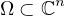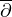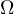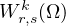Electron. J. Differential Equations, Vol. 2019 (2019), No. 48, pp. 1-22.

Compactness of the canonical solution operator on Lipschitz q-pseudoconvex boundaries Sayed Saber

Abstract:
Letbe a bounded Lipschitz q-pseudoconvex domain that admit good weight functions. We shall prove that the canonical solution operator for the-equation is compact on the boundary ofand is bounded in the Sobolev spacefor some values of k. Moreover, we show that the Bergman projection and the-Neumann operator are bounded in the Sobolev spacefor some values of k. Ifis smooth, we shall give sufficient conditions for compactness of the-Neumann operator.

Submitted May 8, 2018. Published April 10, 2019.
Math Subject Classifications: 35J20, 35J25, 35J60.
Key Words: Lipschitz domain; q-pseudoconvex domain.

Show me the PDF file (407 KB), TEX file for this article.Sayed Saber Mathematics Department Faculty of Science Beni-Suef University, Egypt email: sayedkay@yahoo.com